# Reply Tool - Workflow Engagement Metrics¶

Last Update: 5 January 2020

# Overview¶

The Editing team deployed the reply tool as an opt-out preference on Arabic (arwiki), Czech (cswiki) and Hungarian (huwiki) Wikipedias on 24 September 2020. Further information about the Editing team's work on the tool including the design, deployment process, and status updates is available on the project page. The purpose of this task is to analyze how people are engaging with the new Replying tool to help answer these questions:

• Are people are having success using the feature?
• Are people using the tool in ways that negatively impact the experience of others?

Results of this analysis will be used to determine what - if any - changes should be made to the workflow before deploying the feature to more people and beginning and A/B test to determine its impact.

For this analysis, we reviewed eventlogging data from 24 September 2020 (date of deployment an an opt-out preference) through 08 December 2020. For each metric below, we provided a breakdown by wiki where deployed as opt-out and the users' experience level based on cumulative edits.

In :
library(IRdisplay)

display_html(
'<script>
code_show=true;
function code_toggle() {
if (code_show){
$(\'div.input\').hide(); } else {$(\'div.input\').show();
}
code_show = !code_show
}
$( document ).ready(code_toggle); </script> <form action="javascript:code_toggle()"> <input type="submit" value="Click here to toggle on/off the raw code."> </form>' )  In : shhh <- function(expr) suppressPackageStartupMessages(suppressWarnings(suppressMessages(expr))) shhh({ library(magrittr); library(zeallot); library(glue); library(tidyverse); library(zoo); library(lubridate) library(scales) })  # 1. Are people publishing the replies they start?¶ ## Reply Tool Funnel :¶ We reviewed a sample of data from EditAttemptStep to determine the percent of users that successfully met each step of the reply tool workflow. Specifically, we reviewed the following: • Of the people who click a [ reply ] link (action = 'init'), what % of people see the interface ready (action = 'ready')? • Of the people who see the interface is ready action = 'ready, what % of people start typing a comment (action = 'firstChange')? • Of the people who start typing a comment (action = 'firstChange'), what % of people click the Reply button (action = 'saveIntent')? • Of the people who click the Reply button (action = 'saveIntent'), what % of people successfully publish the comment they were drafting (action = 'saveSuccess')? The data below reflects the number of users not sessions. These are counts of the percentage of unique people who, at some point during the reviewed timeframe between 24 September 2020 and 08 December 2020, that reached a step (e.g. init, ready, firstchange, etc.) in the Reply Tool comment funnel. Anonymous users were excluded from the analysis as we do not track distinct anonymous users. The worflow funnel charts below reflects the percent of all reply tool users that clicked a reply link (reached init) and completed each step to save their comment. The chart is divided to show the workflow funnel for each user experience level based on the number of cumulative edits. In : # collect data on reply tool users workflow completion steps query <- " --only include full sessions where an init was also logged during the timeframe WITH init_sessions AS ( SELECT event.editing_session_id AS session_id, wiki AS wiki FROM event_sanitized.editattemptstep WHERE event.action = 'init' AND year = 2020 AND dt between '2020-09-24' AND '2020-12-08' -- review wikis where deployed as opt-out AND wiki IN ('arwiki', 'cswiki', 'huwiki') AND event.integration= 'discussiontools' -- remove anonymous users AND event.user_id != 0 ) -- review steps completed by each user SELECT user, wiki, min(edit_count) AS edit_count, -- select first editing interface show to user min(editor_interface) AS editor_interface, sum(cast(action = 'init' as int)) >= 1 AS init_completed, sum(cast(action = 'ready' as int)) >= 1 AS ready_completed, sum(cast(action = 'firstChange' as int)) >= 1 AS firstChange_completed, sum(cast(action = 'saveIntent' as int)) >= 1 AS saveIntent_completed, sum(cast(action = 'saveSuccess' as int)) >= 1 AS saveSuccess_completed FROM ( SELECT eas.event.user_editcount AS edit_count, eas.event.user_id AS user, eas.event.editing_session_id AS session_id, eas.event.editor_interface AS editor_interface, eas.event.action AS action, eas.wiki AS wiki, COUNT(*) AS events FROM event_sanitized.editattemptstep eas INNER JOIN init_sessions ON eas.event.editing_session_id = init_sessions.session_id AND eas.wiki = init_sessions.wiki WHERE year = 2020 -- events since deployment date through 08 December 2020 AND dt between '2020-09-24' AND '2020-12-08' -- review wikis where deployed as opt-out AND eas.wiki IN ('arwiki', 'cswiki', 'huwiki') AND eas.event.integration= 'discussiontools' -- remove anonymous users AND eas.event.user_id != 0 GROUP BY eas.event.user_id, eas.event.user_editcount, eas.event.action, eas.event.editor_interface, eas.event.editing_session_id, eas.wiki ) AS sessions_data GROUP BY user, wiki "  In [ ]: collect_reply_workflow_steps <- wmfdata::query_hive(query)  In : # add column with user edit count buckets and by experience level reply_workflow_with_editcount <- collect_reply_workflow_steps %>% mutate(edit_count_bucket = case_when( edit_count == 0 ~ '0 edits', edit_count >=1 & edit_count <= 4 ~ '1-4 edits', edit_count >=5 & edit_count <= 99 ~ '5-99 edits', edit_count >=100 & edit_count <= 999 ~ '100-999 edits', edit_count >=1000 ~ '1000+ edits'), experience_level = case_when( edit_count < 100 ~ 'junior contributors (< 100 cumulative edits)', edit_count > 500 ~ 'senior contributors (> 500 cumulative edits)'))  In : # Order edit counts reply_workflow_with_editcount$edit_count_bucket %<>%
factor(levels= c("0 edits", "1-4 edits", "5-99 edits", "100-999 edits", "1000+ edits"))

In :
reply_users_workflow_funnel <- reply_workflow_with_editcount %>%
group_by(wiki, edit_count_bucket, experience_level, editor_interface) %>%
summarise(init = sum(init_completed == 'true'),
firstchange = sum(firstchange_completed == 'true'),
saveintent = sum(saveintent_completed == 'true'),
savesuccess = sum(savesuccess_completed == 'true'), .groups = 'drop')

In :
# reorganize data so it can be easily charted
gather("step", "n_users", 5:9)

In :
# order edit steps to correspond with the steps of the workflow

In :
# Find total init sessions by edit count bucket across all three target wikis for both interfaces
group_by(edit_count_bucket) %>%
filter(step == 'init')  %>%
summarise(init_users_overall = sum(n_users), .groups = 'drop')

In :
# Find total init sessions across all three target wikis by edit count bucket and editor_interface

group_by(edit_count_bucket, editor_interface) %>%
filter(step == 'init')  %>%
summarise(init_users_byeditcount = sum(n_users), .groups = 'drop')

In [ ]:
# join with workflow table

In [ ]:
# join with workflow table

In :
# Find total init sessions across all three target wikis by editor experience

group_by(experience_level, editor_interface) %>%
filter(step == 'init',
experience_level != 'NA' )  %>% # remove contributors not bucketed into senior or junior levels
summarise(init_users_byexperience = sum(n_users), .groups = 'drop')

In [ ]:
# join with workflow table

In :
# Find total init sessions across all three target wikis by editor experience for both interfaces

group_by(experience_level) %>%
filter(step == 'init',
experience_level != 'NA' )  %>% # remove contributors not bucketed into senior or junior levels
summarise(init_users_byexperience_both = sum(n_users), .groups = 'drop')

In [ ]:
# join with workflow table


## Workflow funnel across all three opt-out wikis by user's experience level¶

In :
# plot workflow across all three target wikis on VE broken down by edit bucket

options(repr.plot.width = 15, repr.plot.height = 10)

filter(editor_interface == 'visualeditor') %>%
group_by(step, edit_count_bucket, editor_interface) %>%
summarise(n_users = sum(n_users),
pct_users = n_users/init_users_byeditcount, .groups = 'drop') %>%
ggplot(aes(x=step, y= pct_users)) +
geom_bar(stat='identity', position = 'dodge', fill = 'darkblue') +
geom_text(aes(label = paste(round(pct_users * 100), '%')), vjust=-0.5) +
geom_text(aes(x,y,label=lab),
data=data.frame(x= 'firstchange', y= 1.05, lab=c("total users: 14","total users: 77","total users: 80", "total users: 32", "total users: 40"),
edit_count_bucket= c("0 edits", "1-4 edits", "5-99 edits", "100-999 edits", "1000+ edits" )), vjust=1) +
facet_grid(~edit_count_bucket) +
scale_y_continuous(labels = scales::percent) +
labs (y = "Percent of reply tool users",
x = "Step",
title = "Visual Editor Reply Tool Workflow Funnel by Editor Experience \n Across All Opt-out Wikis")  +
theme_bw() +
theme(
plot.title = element_text(hjust = 0.5),
text = element_text(size=16),
axis.text.x = element_text(angle=45, hjust=1),
legend.position = "none")

p

ggsave("Figures/ve_reply_workflow_overall_editcount.png", p, width = 16, height = 8, units = "in", dpi = 300)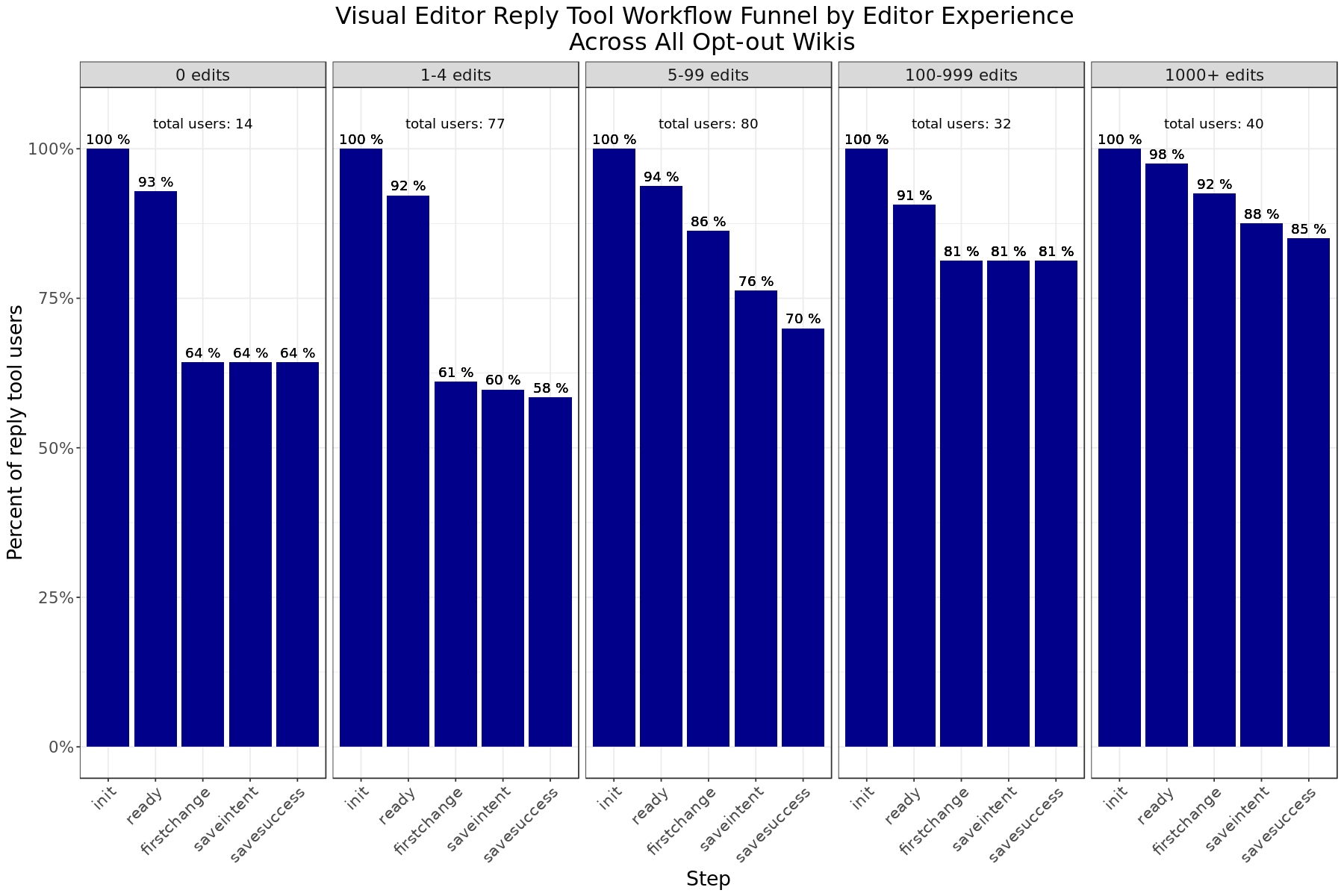In :
# plot workflow across all three target wikis on VE broken down by junior and senior contributors

filter(editor_interface == 'visualeditor') %>%
group_by(step, experience_level, editor_interface) %>%
mutate(n_users = sum(n_users),
pct_users = n_users/init_users_byexperience) %>%
ggplot(aes(x=step, y= pct_users)) +
geom_bar(stat='identity', position = 'dodge', fill = 'darkblue') +
geom_text(aes(label = paste(round(pct_users * 100), '%')), vjust=-0.5) +
geom_text(aes(x,y,label=lab),
data=data.frame(x= 'firstchange', y= 1.05, lab=c("total users: 147","total users: 50"),
experience_level= c("junior contributors (< 100 cumulative edits)", "senior contributors (> 500 cumulative edits)")), vjust=1) +
facet_grid(~experience_level) +
scale_y_continuous(labels = scales::percent) +
labs (y = "Percent of reply tool users",
x = "Step",
title = "Visual Editor Reply Tool Workflow Funnel for Junior and Senior Contributors \n Across All Opt-out Wikis"
)  +
theme_bw() +
theme(
plot.title = element_text(hjust = 0.5),
text = element_text(size=16),
legend.position = "none")

p

ggsave("Figures/ve_reply_workflow_overall_experience.png", p, width = 16, height = 8, units = "in", dpi = 300)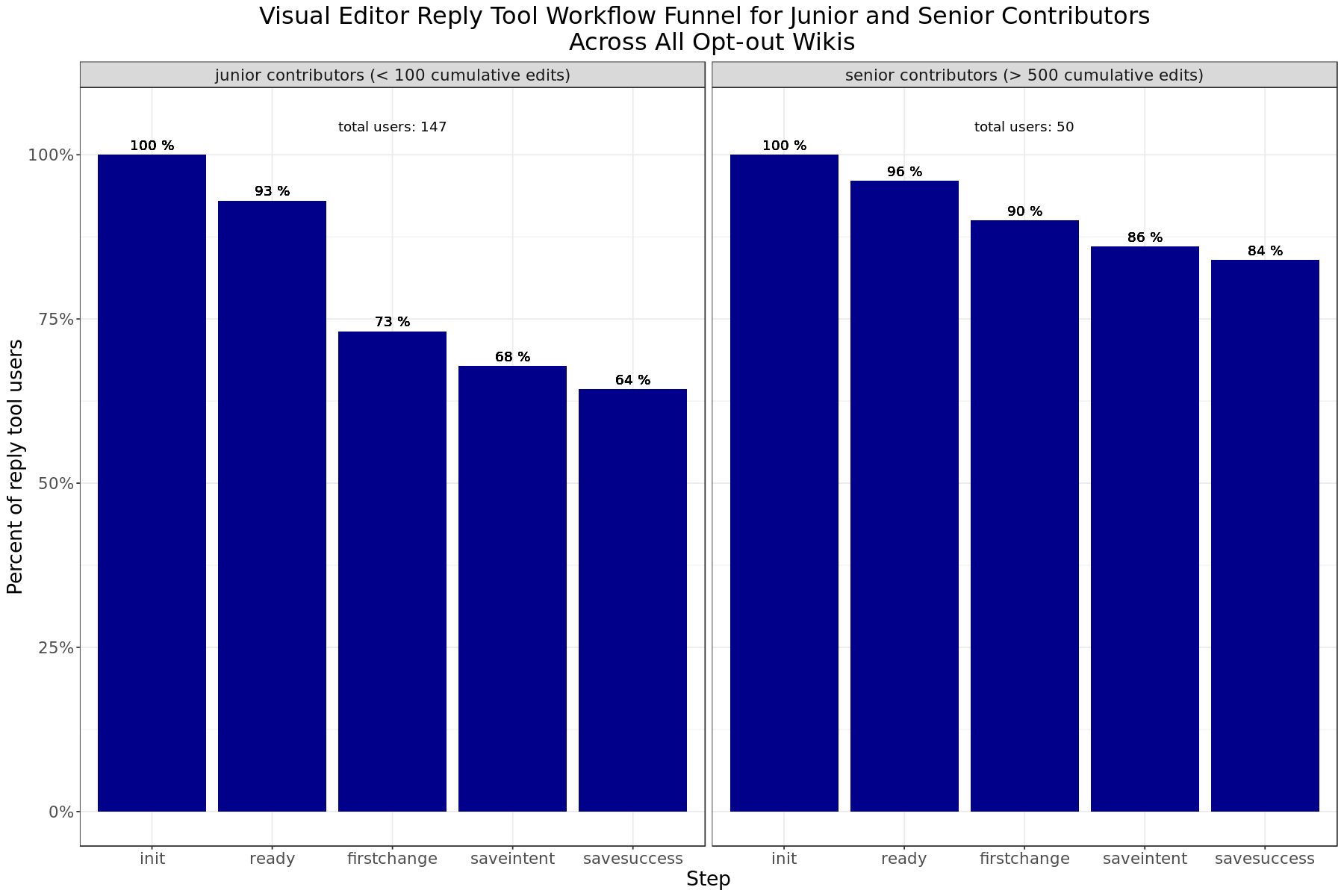Across all three opt-out wikis, there is only a slight drop in the number of senior contributors that complete each step of the reply workflow using visual editor with 84% of all senior contributors sucessfully completing their edit. The largest decrease occurs between init and ready which may be due to network disruptions during the visual editor load time.

For junior contributors, the biggest drop in number of contributors occurs between ready (the contributor clicks the reply link and the editing interface is ready) and firstchange (the user begins typing a comment). However, the rate of drop-off decreases after this step. While only 79% percent of junior contributors that successfully loaded the editing interface started typing a comment, 93% percent of junior contributors that started typing a comment were able to successuly save their comment.

Interestingly, reply tool users with 5 to 99 cumulative had the highest drop-off between firstchange and saveintent steps.

In :
# plot workflow across all three target wikis on wikitext broken down by experience

filter(editor_interface == 'wikitext') %>%
group_by(step, edit_count_bucket, editor_interface) %>%
mutate(n_users = sum(n_users),
pct_users = n_users/init_users_byeditcount) %>%
ggplot(aes(x=step, y= pct_users)) +
geom_bar(stat='identity', position = 'dodge', fill = 'red4') +
geom_text(aes(label = paste(round(pct_users * 100), '%')), vjust=-0.5) +
geom_text(aes(x,y,label=lab),
data=data.frame(x= 'firstchange', y= 1.05, lab=c("total users: 4","total users: 31","total users: 52", "total users: 25", "total users: 114"),
edit_count_bucket= c("0 edits", "1-4 edits", "5-99 edits", "100-999 edits", "1000+ edits" )), vjust=1) +
facet_grid(~edit_count_bucket) +
scale_y_continuous(labels = scales::percent) +
labs (y = "Percent of reply tool users",
x = "Step",
title = "Wikitext Reply Tool Workflow Funnel by Editor Experience \n Across All Opt-Out Wikis")  +
theme_bw() +
theme(
plot.title = element_text(hjust = 0.5),
text = element_text(size=16),
axis.text.x = element_text(angle=45, hjust=1),
legend.position = "none")

p

ggsave("Figures/wikitext_reply_workflow_overall_editcount.png", p, width = 16, height = 8, units = "in", dpi = 300)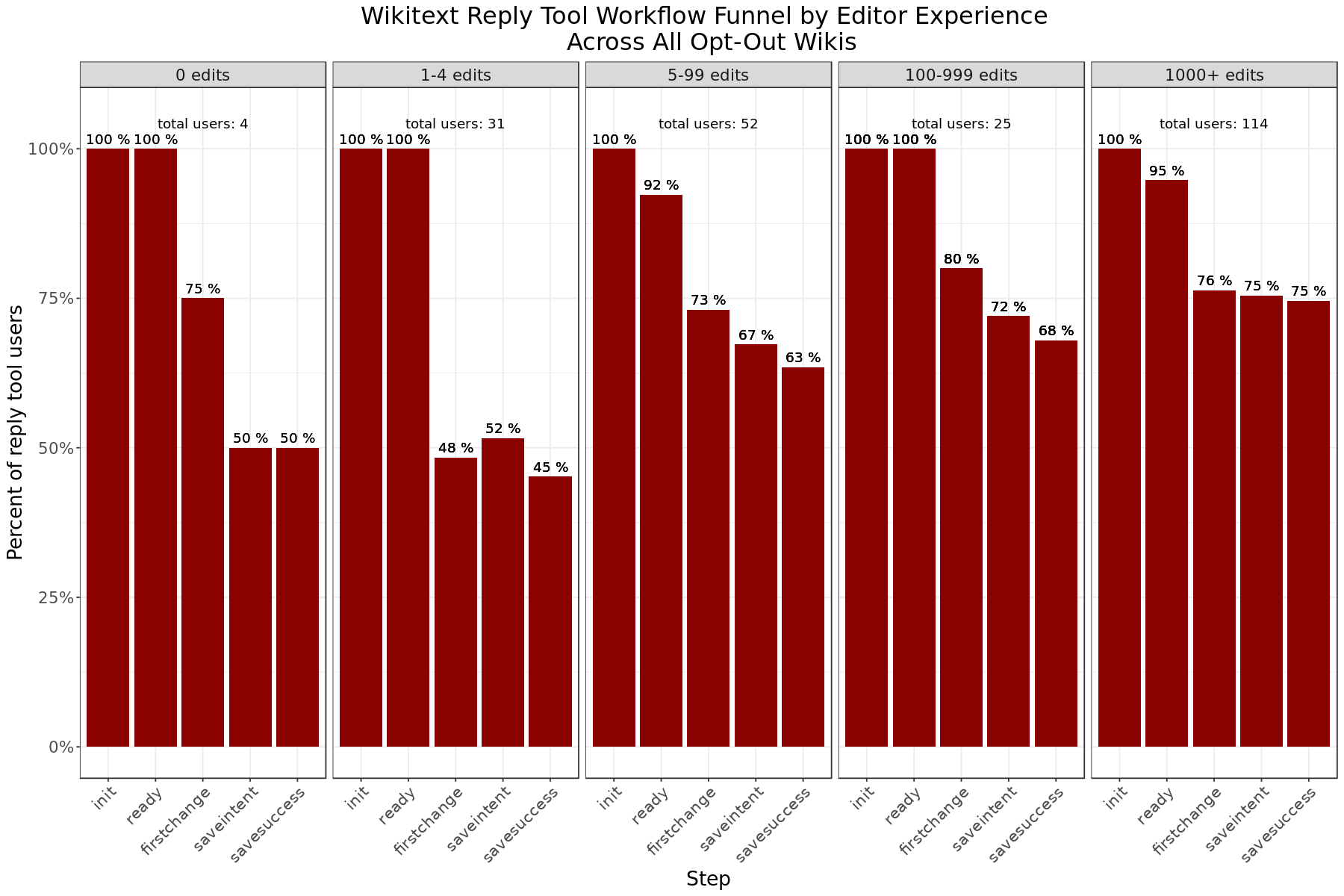In :
# plot workflow across all three target wikis on VE broken down by junior and senior contributors

filter(editor_interface == 'wikitext') %>%
group_by(step, experience_level, editor_interface) %>%
mutate(n_users = sum(n_users),
pct_users = n_users/init_users_byexperience) %>%
ggplot(aes(x=step, y= pct_users)) +
geom_bar(stat='identity', position = 'dodge', fill = 'red4') +
geom_text(aes(label = paste(round(pct_users * 100), '%')), vjust=-0.5) +
geom_text(aes(x,y,label=lab),
data=data.frame(x= 'firstchange', y= 1.05, lab=c("total users: 87","total users: 117"),
experience_level= c("junior contributors (< 100 cumulative edits)", "senior contributors (> 500 cumulative edits)")), vjust=1) +
facet_grid(~experience_level) +
scale_y_continuous(labels = scales::percent) +
labs (y = "Percent of reply tool users",
x = "Step",
title = "Wikitext Reply Tool Workflow Funnel for Junior and Senior Contributors \n Across All Opt-out Wikis"
)  +
theme_bw() +
theme(
plot.title = element_text(hjust = 0.5),
text = element_text(size=16),
axis.text.x = element_text(angle=45, hjust=1),
legend.position = "none")

p

ggsave("Figures/wikitext_reply_workflow_overall_experience.png", p, width = 16, height = 8, units = "in", dpi = 300)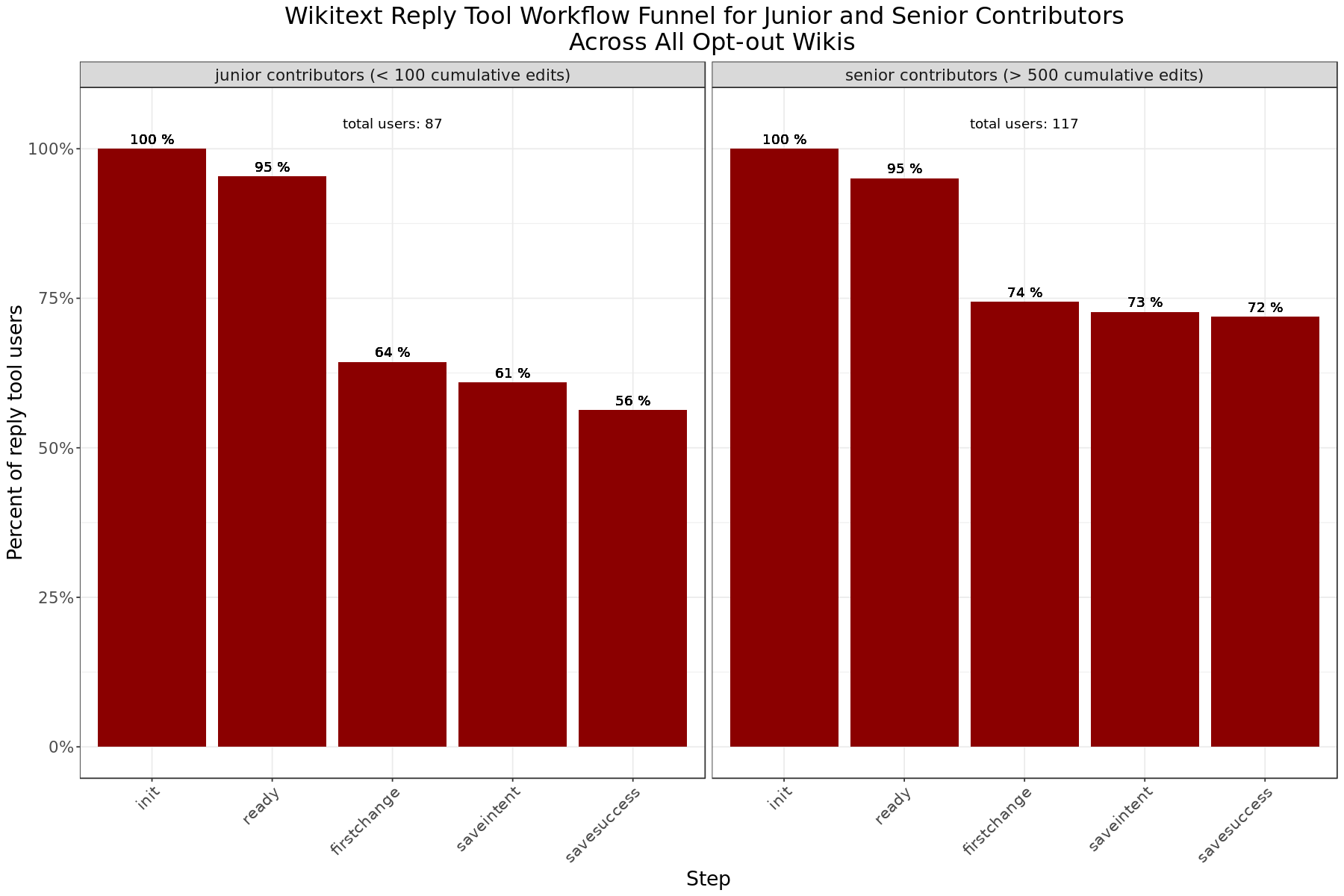Compared to Visual Editor, a smaller percentage of contributors users that successuly load the editing interface (step = ready) start typing a comment (step = firstChange) using wikitext. For editors with 1 to 4 cumulative edits, only 48% of users that clicked the reply link and reached ready started typing a comment.

## Workflow funnel for each opt-out wikis by user's experience level¶

We also reviewed the worflow funnel for each individual wiki to confirm any differences on a per wiki basis.

In :
# Find total init sessions for each target wiki by editcount

group_by(edit_count_bucket, editor_interface, wiki) %>%
filter(step == 'init')  %>%
summarise(init_users_editcount = sum(n_users), .groups = 'drop')

In [ ]:
# join with workflow table

In :
# Find total init sessions for each target wiki by experience level

group_by(experience_level, editor_interface, wiki) %>%
filter(step == 'init',
experience_level != 'NA')  %>%
summarise(init_users_experience = sum(n_users), .groups = 'drop')

In [ ]:
# join with workflow table

In :
# plot workflow on arwiki on VE broken down by edit count

filter(wiki == 'arwiki',
editor_interface == 'visualeditor') %>%
group_by(step, edit_count_bucket, editor_interface) %>%
mutate(n_users = sum(n_users),
pct_users = n_users/init_users_editcount) %>%
ggplot(aes(x=step, y= pct_users)) +
geom_bar(stat='identity', position = 'dodge', fill = 'darkblue') +
geom_text(aes(label = paste(round(pct_users * 100), '%')), vjust=-0.5) +
geom_text(aes(x,y,label=lab),
data=data.frame(x= 'firstchange', y= 1.1, lab=c("total users: 10","total users: 29","total users: 34", "total users: 14", "total users: 25"),
edit_count_bucket= c("0 edits", "1-4 edits", "5-99 edits", "100-999 edits", "1000+ edits" )), vjust=1) +
facet_grid(~edit_count_bucket) +
scale_y_continuous(labels = scales::percent) +
labs (y = "Percent of reply tool users",
x = "Step",
title = "Visual Editor Reply Tool Workflow Funnel by Editor Experience \n on Arabic Wikipedia ")  +
theme_bw() +
theme(
plot.title = element_text(hjust = 0.5),
text = element_text(size=16),
axis.text.x = element_text(angle=45, hjust=1),
legend.position = "none")

p

ggsave("Figures/ve_reply_workflow_arwiki_editcount.png", p, width = 16, height = 8, units = "in", dpi = 300)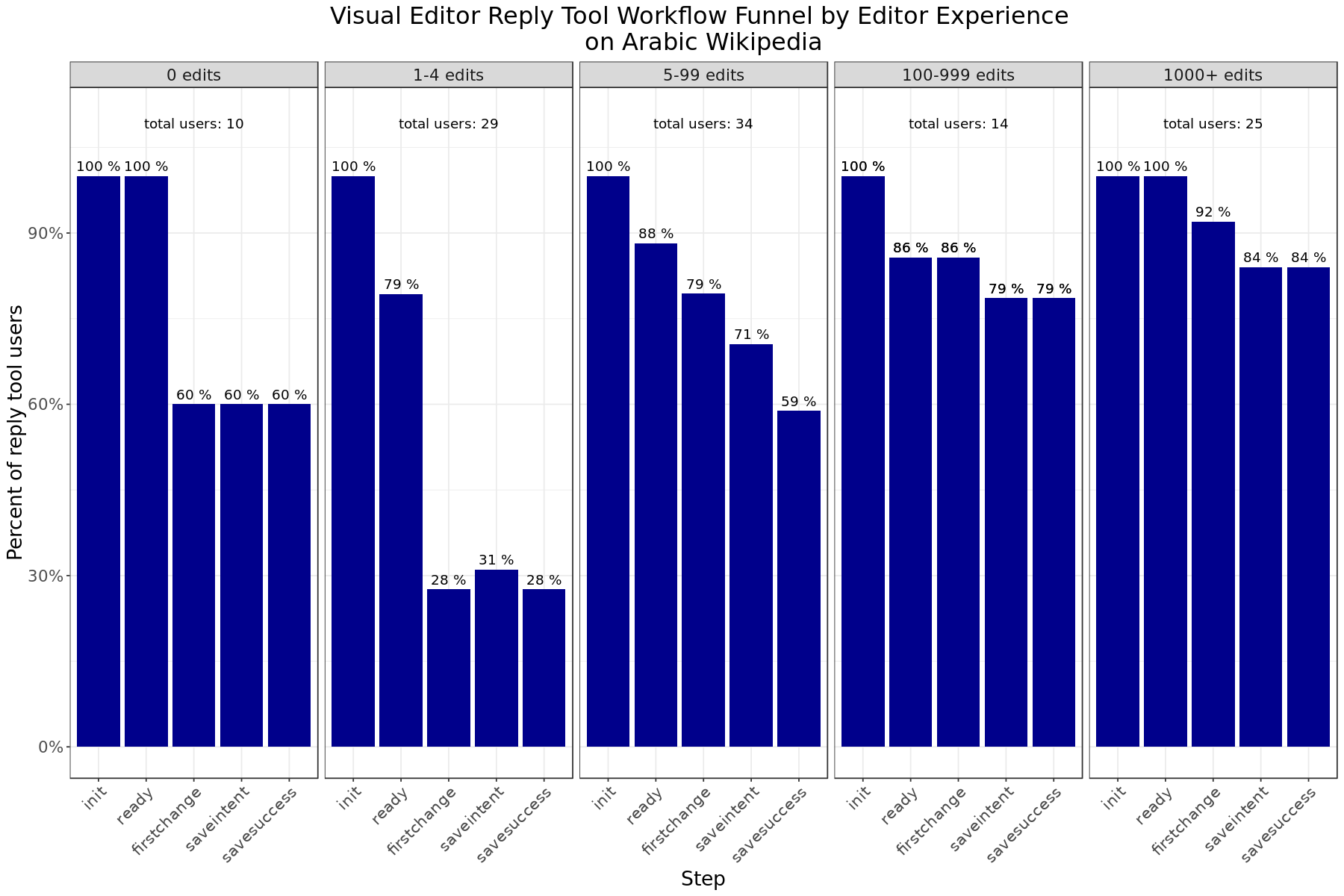In :
# plot workflow on arwiki on VE broken down by experience

filter(wiki == 'arwiki',
editor_interface == 'visualeditor') %>%
group_by(step, experience_level, editor_interface) %>%
mutate(n_users = sum(n_users),
pct_users = n_users/init_users_experience) %>%
ggplot(aes(x=step, y= pct_users)) +
geom_bar(stat='identity', position = 'dodge', fill = 'darkblue') +
geom_text(aes(label = paste(round(pct_users * 100), '%')), vjust=-0.5) +
geom_text(aes(x,y,label=lab),
data=data.frame(x= 'firstchange', y= 1.1, lab=c("total users: 73","total users: 29"),
experience_level = c("junior contributors (< 100 cumulative edits)", "senior contributors (> 500 cumulative edits)")), vjust=1) +
facet_grid(~experience_level) +
scale_y_continuous(labels = scales::percent) +
labs (y = "Percent of reply tool users",
x = "Step",
title = "Visual Editor Reply Tool Workflow Funnel by Junior and Senior Contributors \n on Arabic Wikipedia ")  +
theme_bw() +
theme(
plot.title = element_text(hjust = 0.5),
text = element_text(size=16),
axis.text.x = element_text(angle=45, hjust=1),
legend.position = "none")
p

ggsave("Figures/ve_reply_workflow_arwiki_experience.png", p, width = 16, height = 8, units = "in", dpi = 300)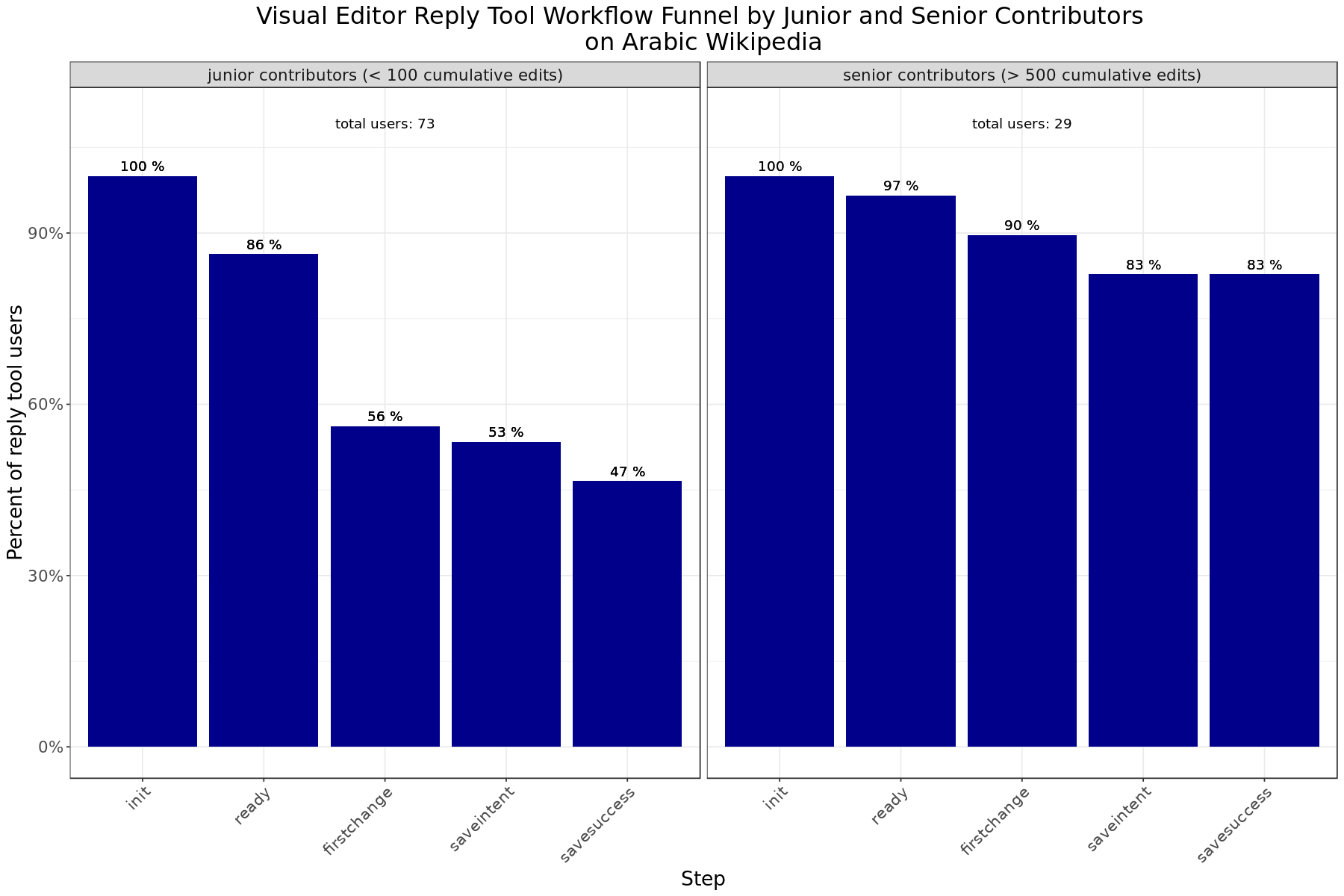In :
# plot workflow on arwiki on wikitext broken down by experience

filter(wiki == 'arwiki',
editor_interface == 'wikitext') %>%
group_by(step, edit_count_bucket, editor_interface) %>%
mutate(n_users = sum(n_users),
pct_users = n_users/init_users_editcount) %>%
ggplot(aes(x=step, y= pct_users)) +
geom_bar(stat='identity', position = 'dodge', fill = 'red4') +
geom_text(aes(label = paste(round(pct_users * 100), '%')), vjust=-0.5) +
geom_text(aes(x,y,label=lab),
data=data.frame(x= 'firstchange', y= 1.1, lab=c("total users: 3","total users: 20","total users: 34", "total users: 9", "total users: 31"),
edit_count_bucket= c("0 edits", "1-4 edits", "5-99 edits", "100-999 edits", "1000+ edits" )), vjust=1) +
facet_grid(~edit_count_bucket) +
scale_y_continuous(labels = scales::percent) +
labs (y = "Percent of reply tool users",
x = "Step",
title = "Wikitext Reply Tool Workflow Funnel by Editor Experience \n on Arabic Wikipedia")  +
theme_bw() +
theme(
plot.title = element_text(hjust = 0.5),
text = element_text(size=16),
axis.text.x = element_text(angle=45, hjust=1),
legend.position = "none")

p

ggsave("Figures/wikitext_reply_workflow_arwiki_editcount.png", p, width = 16, height = 8, units = "in", dpi = 300)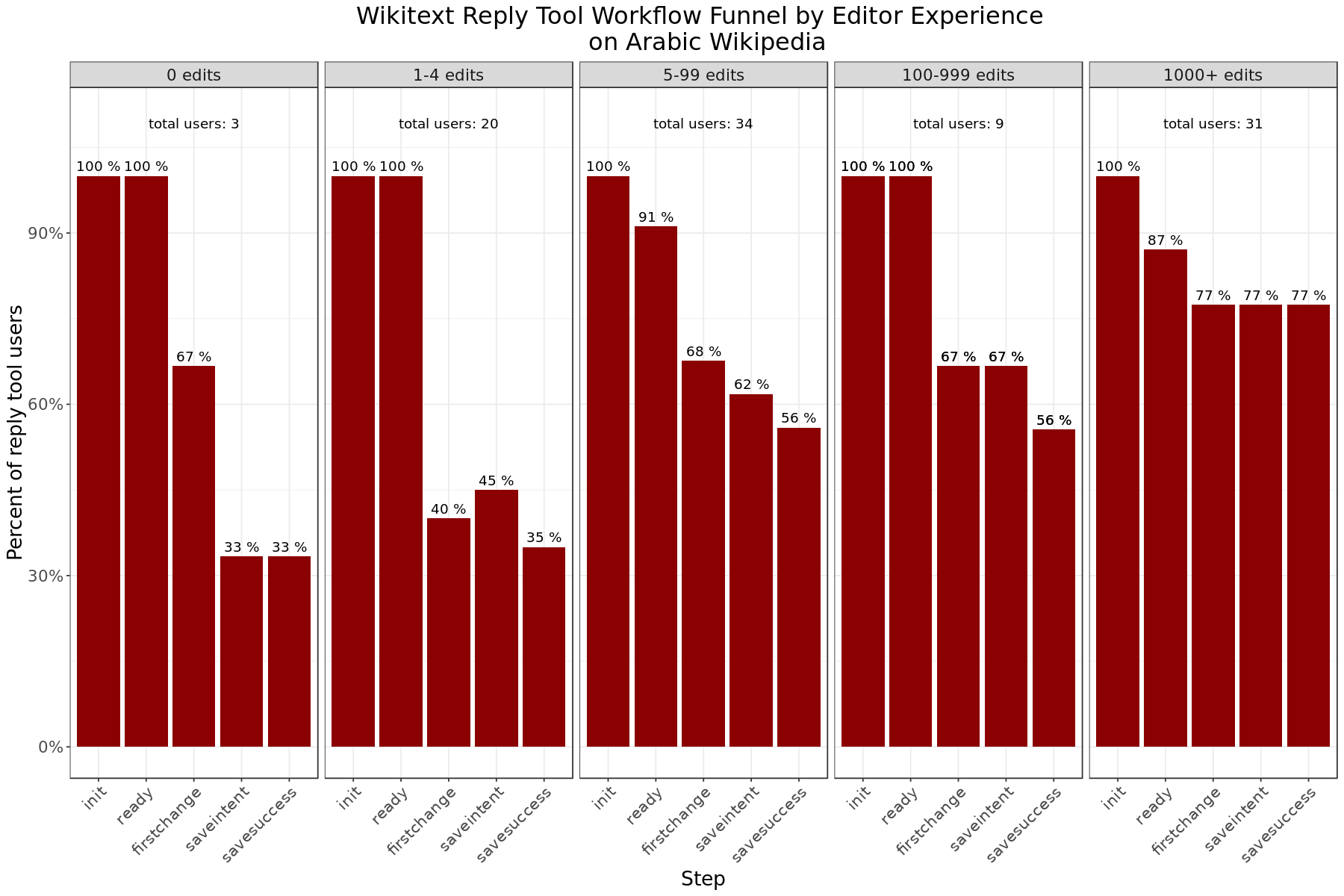In :
# plot workflow on arwiki on VE broken down by experience

filter(wiki == 'arwiki',
editor_interface == 'wikitext') %>%
group_by(step, experience_level, editor_interface) %>%
mutate(n_users = sum(n_users),
pct_users = n_users/init_users_experience) %>%
ggplot(aes(x=step, y= pct_users)) +
geom_bar(stat='identity', position = 'dodge', fill = 'red4') +
geom_text(aes(label = paste(round(pct_users * 100), '%')), vjust=-0.5) +
geom_text(aes(x,y,label=lab),
data=data.frame(x= 'firstchange', y= 1.1, lab=c("total users: 73","total users: 29"),
experience_level = c("junior contributors (< 100 cumulative edits)", "senior contributors (> 500 cumulative edits)")), vjust=1) +
facet_grid(~experience_level) +
scale_y_continuous(labels = scales::percent) +
labs (y = "Percent of reply tool users",
x = "Step",
title = "Wikitext Reply Tool Workflow Funnel by Junior and Senior Contributors \n on Arabic Wikipedia ")  +
theme_bw() +
theme(
plot.title = element_text(hjust = 0.5),
text = element_text(size=16),
axis.text.x = element_text(angle=45, hjust=1),
legend.position = "none")
p

ggsave("Figures/wikitext_reply_workflow_arwiki_experience.png", p, width = 16, height = 8, units = "in", dpi = 300)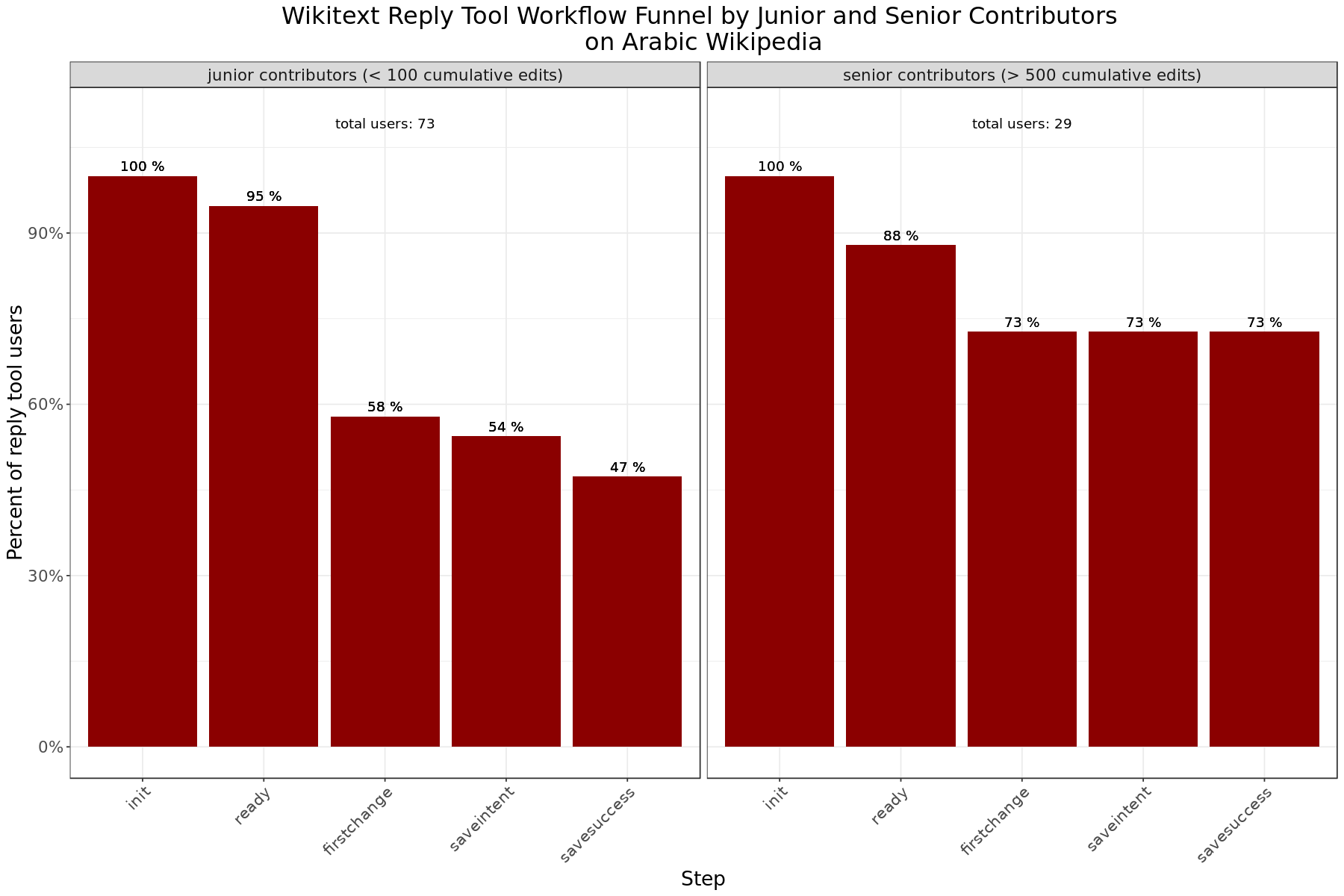In :
# plot workflow on cswiki on VE broken down by experience

filter(wiki == 'cswiki',
editor_interface == 'visualeditor') %>%
group_by(step, edit_count_bucket, editor_interface) %>%
mutate(n_users = sum(n_users),
pct_users = n_users/init_users_editcount) %>%
ggplot(aes(x=step, y= pct_users)) +
geom_bar(stat='identity', position = 'dodge', fill = 'darkblue') +
geom_text(aes(label = paste(round(pct_users * 100), '%')), vjust=-0.5) +
geom_text(aes(x,y,label=lab),
data=data.frame(x= 'firstchange', y= 1.1, lab=c("total users: 4","total users: 18","total users: 24", "total users: 9", "total users: 8"),
edit_count_bucket= c("0 edits", "1-4 edits", "5-99 edits", "100-999 edits", "1000+ edits" )), vjust=1) +
facet_grid(~edit_count_bucket) +
labs (y = "Percent of reply tool users",
x = "Step",
title =  "Visual Editor Reply Tool Workflow Funnel by Editor Experience \n on Czech Wikipedia")  +
theme_bw() +
theme(
plot.title = element_text(hjust = 0.5),
text = element_text(size=16),
axis.text.x = element_text(angle=45, hjust=1),
legend.position = "none")

p

ggsave("Figures/ve_reply_workflow_cswiki_editcount.png", p, width = 16, height = 8, units = "in", dpi = 300)